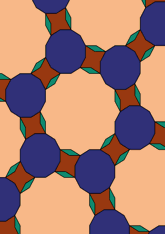# Derived from 4.6.12, with diamonds and 12-gondata145/D4612C

## Geometry

• The symmetry group of the tiling is *632 (p6m).
• All the internal angles of the constituent polygons are a multiple of 30°.
• Contains one regular two-pointed star polygon with vertex angle of 30°.
• Contains one regular four-pointed star polygon with vertex angle of 60°.
• Contains one regular 12-gon.
• Contains one regular 12-pointed star polygon with vertex angle of 120°.
• The tiling satisfies the two-colour condition.
• The tiling is edge-to-edge.
• As drawn, contains about 95 polygons.

## References

Publications referenced:
1. Derived from 4.6.12 of B GrÃ¼nbaum and G C Shephard. Tilings and Patterns, W H Freeman, 1987. ISBN 071671193. [gands] {Most important work in this area.}
2. Uniform Star Polygons with Digons of B A Wichmann. The World of Patterns, World Scientific, 2001. ISBN 981024619. [wich2] {Only new patterns ascribed to this.}

v53